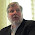## Monday, June 30, 2014

### Characterizing Airborne Particulate Exposure (particle size)

In the last blog I briefly introduced the general topic of aerosol science as it relates to human health exposure and risk assessment with a focus on insoluble particulate.   The point was made that aerosol particles even if they are identical in composition and come from the same source will typically be comprised of various sizes and sometimes different shapes.   We also saw that the size of any individual particle – expressed as an aerodynamic diameter (AD) – determines its probability of being inhaled and the where in the respiratory track it could be deposited.

This week’s blog goes into more detail as to how we characterize the particle sizes within any real aerosol.

Let us consider a relatively fine powder of unit density in which the vast majority of particles are between 150-200 micrometers  AD.    You might not expect this powder to be very “dusty” if you were to suspend it in a workroom air volume.    Indeed, particles this big would tend to settle to the floor within a few seconds.   However, what if the material was being conveyed by pneumatic transport from a large bin to bags?   If the rate of transfer was high enough, even a relatively small percentage of “fines” or particles within the powder much smaller than 100 microns AD  could contribution strongly to a persistent airborne aerosol in the workroom.   Add to this the critical fact that the particles tend to break apart (are friable) on impact with the conveyor walls and with each other and this would dramatically add to the aerosol exposure potential.

In this case, we need to sample the air to see exactly what is going on with the airborne particulate concentration and the range of sizes. Today we have some remarkable optical devises to assist us; however, in the old days we did this with an Anderson Impactor which involved putting papers or other impacting surfaces on a series of impaction plates in the device with looks like a cylindrical coffee-maker.   We would draw enough air through the unit and the particles would be deposited on the various stages of the impactor depending on their specific range of AD.    One could then measure the relative weight in all the stages and calculate/model an actual distribution for the ADs of all the particles.   If I get more than a few folks asking me to go into the details of this process I will happily present it in a future blog.   For now we will just use the results.

The end result is that we find that the distribution of ADs is well described by a log-normal distribution of the total airborne mass. The parameters of a lognormal aerosol mass distribution are

1: The mass median aerodynamic diameter (MMAD)
2.  the Geometric Standard Deviation or GSD.

The MMAD is the mediam particle size (half the mass is in particles smaller and half in larger than this size) and the GSD is the ratio of the 84th percentile and the 50% (median) percentile sizes.    Graphs of a real-world aerosol distribution from some of my previous in-plant research are presented below:

The above is a Lognormal Distribution generated using MMAD = 10 microns and GSD = 2

The graph above is the log of the AD size versus percentage on a probit scale

You can see from the top graph that the distribution (like all log-normal distributions) is bounded by zero and skewed toward lower values.   This is because gravity favors keeping the smaller particles in the air longer than the large particles.

As you travel along this distribution you can see the relative contributions to the distribution of the various ADs and therein lays the real value of this analysis.  For example, particles with AD = 2 and smaller represent about 1% of the total aerosol mass in this example. You can also inspect this visually to get some idea as to how much of the total mass of this distribution is in particulate 10 microns AD and lower.  This is, the portion of the total mass of the aerosol that has some reasonable chance of making it to the deep or pulmonary regions of the lungs.

Using the parameters of this distribution and the ACGIH mathematical relationship listed for respirable mass, one can estimate the exact percentage of the total airborne particulate this is indeed respirable.   In this example, it was about 12%.  This is exactly what respirable mass cyclone personal samplers are supposed to do.    That is if you sampled the example aerosol for total airborne mass concentration and got 10 mg/m3, a concurrent respirable mass sample should come in around 1.2 mg/m3.

This blog was designed simply to provide you with some insight into the characterization of airborne particulate mass as a distribution.    If more than a few of you would like me to go into some of the computational details and spreadsheet software, I would be happy to do so in a future blog.

1.This is good stuff! Thanks.

2.I would be interestd in computational details and spreadsheet software. Thank you!

3.Great. I have a vote of one. A few more and I will put the details into a blog.
Many thanks, Mike

4.Thank you Mike -I'd hate to get embroiled in Log Normal discussions again so I won't.

5.